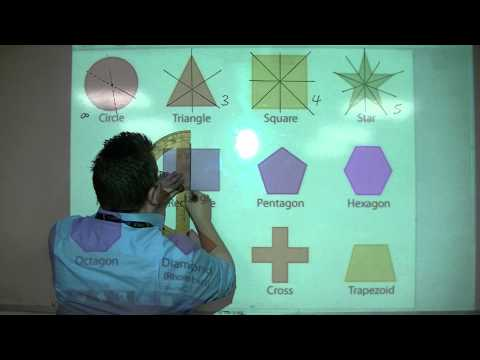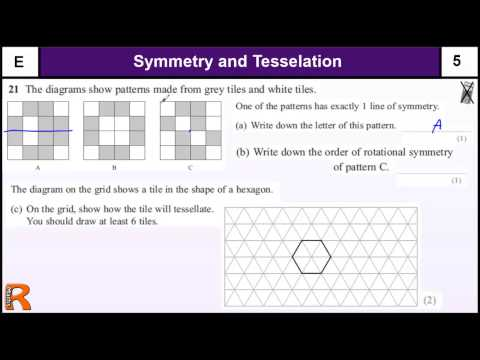## Symmetry, Design and Tessellations

Subject: Compulsory Maths

#### Overview

This note gives information about tessellation and its types and also it gives information about symmetry.A tessellation is known as tiling .
##### Symmetry, Design and Tessellations

The dotted line which is the half part of each shape to one side of a dotted line is the mirror image of the other parts is called the line of symmetry . In another word, it is also called the axis of symmetry , so the figure is said to have bilateral symmetry or to be symmetrical by reflection in the dotted line and a line of symmetry shape can be turned so as it exactly into its outline.

Rotational symmetry

When a shape can be mapped onto itself by a rotation of less than a complete turn (360o) about a point the shape is said to have a rotational symmetry .

Order of rotational symmetry

When the shape is rotated through a half -turn it can be fitted to the original shape . It means , when it is rotated through 2 times half-turns , it reaches its original position .

$\therefore$ It has rotational symmetry of order 2.

When the equilateral triangle is rotated through a $\frac{1}{3}$ of turn 120o, it can fit over the original shape. When it is rotated through3 times $\frac{1}{3}$ and then it will reach its original place.

$\therefore$ It has rotational symmetry of order 3.

When the square is rotated through quarter-turn $\frac{1}{4}$of the turn 90o. Which can be fitted over the original shape . It means when it is rotated through 4time $\frac{1}{4}$of turn , it reaches its original position .

$\therefore$ It has rotational symmetry of order 4.

Tessellation

A tessellation is known as tiling . It is covering of the plane with congruent geometrical shape un which a repeating pattern without leaving any gaps and without overlapping each other.The shape is polygons in it . It may be an equilateral triangle , regular hexagon or square . To make a tessellation :

• We should not leave any gaps.
• We should not have any overlaps.
• We should use the sets of congruent figures .

Types of tessellation

• Irregular tessellation : In this case , we use irregular types of polygons.
• Semi-regular tessellations:In this case , we use two or more regular polygons . In the adjoining tessellation , regular octagons and squares are used .
• Regular tesselation : In this case, we use the same types of regular polygons . The polygons that we use may be equilateral triangle, regular hexagon etc.

##### Things to remember
• When the shape is rotated through a half -turn it can be fitted to the original shape .
• To make a tessellation we should use the sets of congruent figures .
• A tessellation is known as tiling .
• It includes every relationship which established among the people.
• There can be more than one community in a society. Community smaller than society.
• It is a network of social relationships which cannot see or touched.
• common interests and common objectives are not necessary for society.
##### Videos for Symmetry, Design and Tessellations##### GCSE Maths from Scratch 18.05a Lines of Symmetry